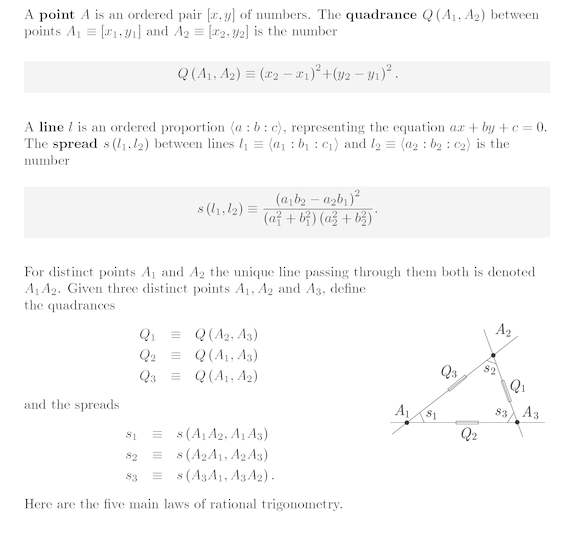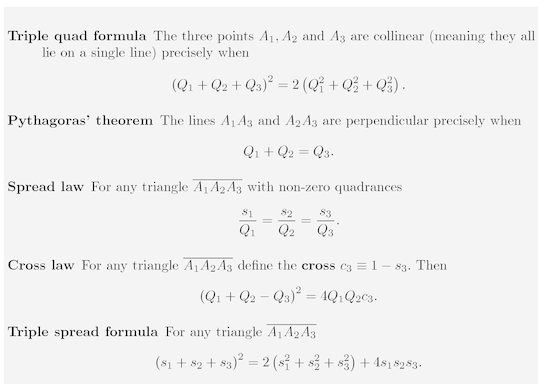# Book: UniversalHyperbolicGeometry

Write down all of the results of Universal Hyperbolic Geometry. Organize them and interpret them in terms of

• matrices and symmetric functions of eigenvalues
• Lie theory (Lie bracket, Jacobi identity...)

Affine geometry

Lines are parallel:

• All (n 2) slopes the same.
• Collinearity: Triple quad formula

Affine combinations

Vector is the relation between parallel lines.

Projective geometry

Space is divided into "infinity" and "finity".

Conformal geometry

Lines are perpendicular: Pythagorean theorem, inner product

Quadratic interpolations: law of cosines A2 - 2ABcos(theta) + B2 = C2 interpolation of (A-B)2 = C2, A2 + B2 = C2, (A+B)2 = C2. Consider triangles more generally. Or consider the function: A2 + B2 - C2 which is 0 when A and B are perpendicular, and nonzero 2ABcos(theta) otherwise.

Inner product defines the equation of a line and whether points lie on it. (a1, a2, a3)(x1, x2, 1) = 0.

Symplectic geometry

Oriented area, volume given by determinant.

• Quadrea: 4 x determinant squared. Determinant is positive three cycle and negative three cycle.
• Quadrea: Archimedes function (and when zero, we have collinearity by the Triple quad formula.
• Quadrea: Can repeatedly factor Archimedes function as a2-b2 = (a+b)(a-b) to get Heron's law for the area in terms of the lengths of the edges.What if we interpret the line as ax + by + cz = 0 where z=1 ?Why is the s1s2s3 term of the third power and not the second power?

Parsiųstas iš http://www.ms.lt/sodas/Book/UniversalHyperbolicGeometry
Puslapis paskutinį kartą pakeistas 2018 vasario 04 d., 11:34